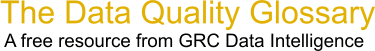Choose Index below for a list of all words and phrases defined in this glossary.

# Boxplot

index | Index

## Boxplot - definition(s)

Boxplot - A box plot, also known as a box and whisker diagram, is a basic graphing tool that displays centering, spread, and distribution of a continuous data set.

A box and whisker plot provides a 5 point summary of the data.

```   1) The box represents the middle 50% of the data.
2) The median is the point where 50% of the data is above it and 50% below it. (Or left and right depending on orientation).
3) The 25th quartile is where, at most, 25% of the data fall below it.
4) The 75th quartile is where, at most, 25% of the data is above it.
5) The whiskers cannot extend any further than 1.5 times the length of the inner quartiles. If you have data points outside this they will show up as outliers.
```

[Category=Data Quality ]

Source: iSixSigma, 02 January 2011 09:58:16, http://www.isixsigma.com/index.php?option=com_glossaryData Quality Glossary.  A free resource from GRC Data Intelligence. For comments, questions or feedback: dqglossary@grcdi.nl